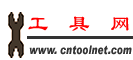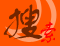工具搜索引擎 加入收藏 意见反馈 帮助中心 繁體中文 资讯标题 资讯内容 商业机会 商机简介 公司名称 公司简介 超市名称 超市简介
 首页 工具超市 产品信息 资讯中心 工具黄页 站长工具 实用工具 会员管理 联系我们

# 过程 (Procedure) - 子例程 (Sub) 和函数 (Function)

## 什么是过程 (Procedure)

Visual Basic 2005 提供了很多内置过程来执行常见的操作，但是总会有一些情况，你希望程序执行内置过程无法处理的操作。这时候，你就需要自己编写过程来完成此任务。另外，自己编写过程也有利于你代码的复用。

## 子例程 (Sub)

`Private Sub ShowYearOfNow`
`Dim theDate As Date = Now`
`MessageBox.Show(theDate.Year, "Show Year")`
`End Sub`

`Public Class Form1`
`    Private Sub btnCall_Click(ByVal sender As System.Object, _`
`    ByVal e As System.EventArgs) Handles btnCall.Click`
`        ShowYear()`
`    End Sub`
` `
`    Private Sub ShowYear()`
`        Dim theDate As Date = Now`
`        MessageBox.Show(theDate.Year, "Show Year")`
`    End Sub`
` `
`End Class`

`    Private Sub btnCall_Click(ByVal sender As System.Object, _`
`    ByVal e As System.EventArgs) Handles btnCall.Click`
`        Dim theDate As Date = Now`
`        MessageBox.Show(theDate.Year, "Show Year")`
`   End Sub`

## 函数 (Function)

`Public Class Form1`
` `
`    Private Sub btnCall_Click(ByVal sender As System.Object, _`
`    ByVal e As System.EventArgs) Handles btnCall.Click`
`        Dim theYear As Integer`
`        theYear = GetYear()`
`        MessageBox.Show(theYear, "Show Year")`
`    End Sub`
` `
`    Private Function GetYear() As Integer`
`        Dim theDate As Date = Now`
`        Return theDate.Year`
`    End Function`
`End Class`

## Sub 和 Function 中的参数

`    Private Function GetArea(ByVal length As Integer, _`
`    ByVal width As Integer) As Integer`
`        Return length * width`
`    End Function`

###### VB 2005 入门教程

If...Then...Else 条件语句
Select...Case 条件语句
For...Next 循环语句
 暂无相关评论

 发表评论： 用户: 验证码

 版权所有 中国工具网 电话：0523-86864621